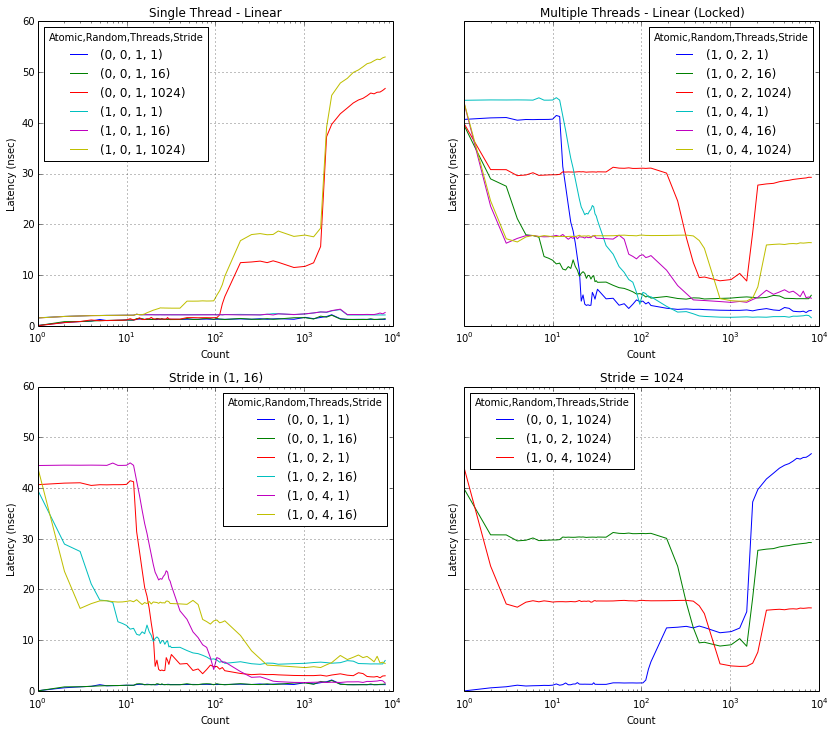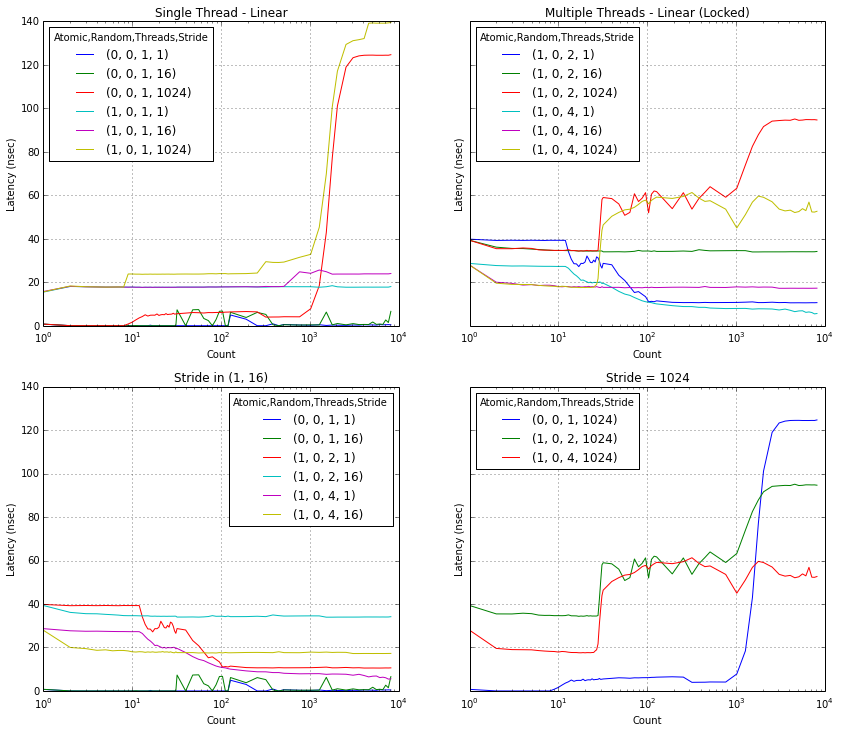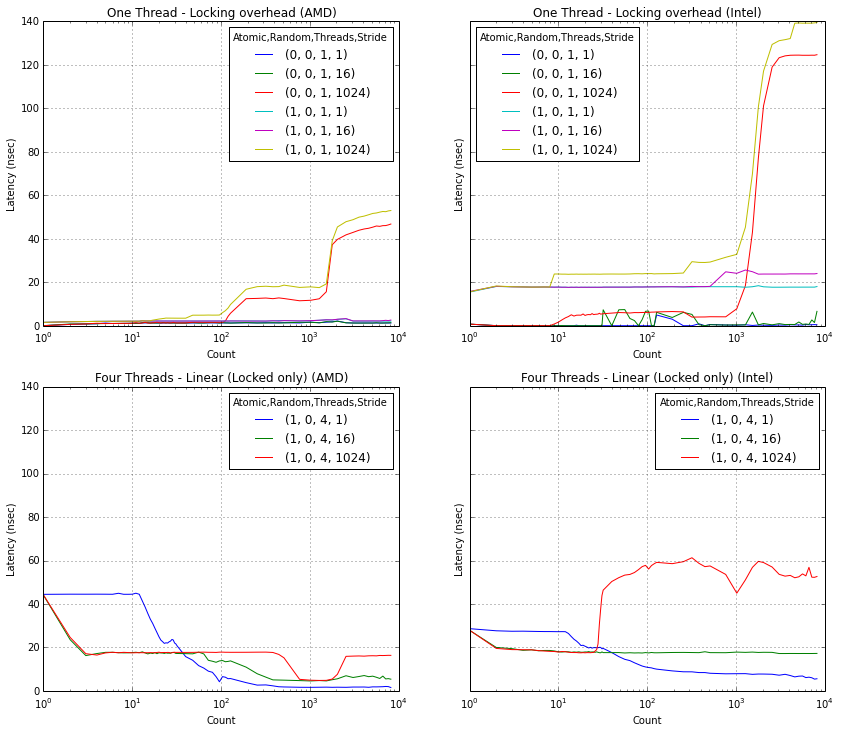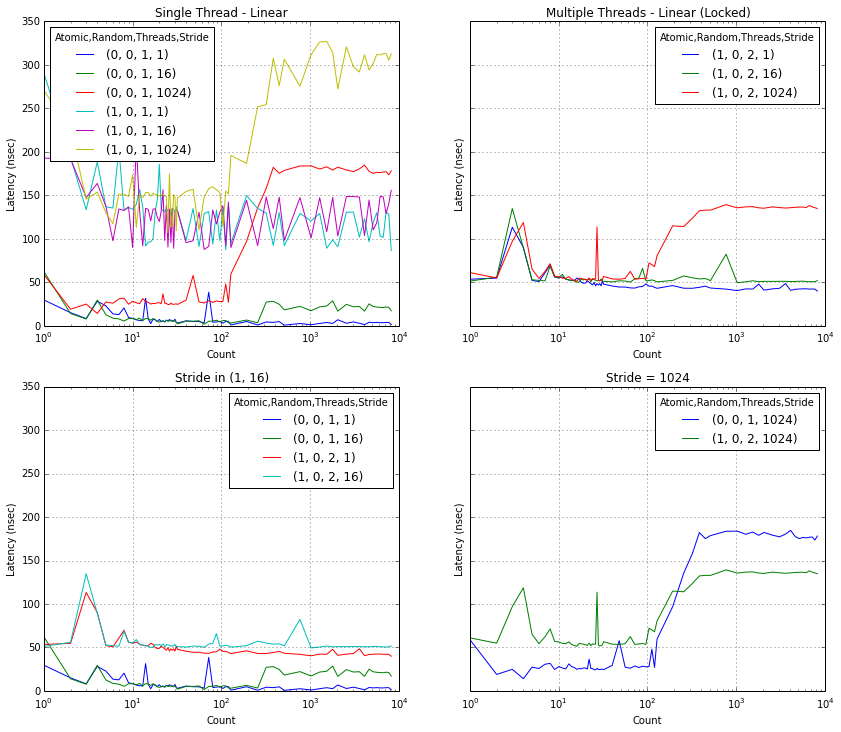# Atomic Operations¶

This is my analysis of results generated from my atomic increment microbenchmark. You should checkout the readme there before reading the rest of this page.

My primary focus was on low-level, low-concurrency (1-4 threads) performance on mainstream processors (2-6 cores).

I started the analysis by loading the data (generated with make quickreport) into Pandas:

In :
import pandas as pd

data_1045T = pd.read_csv('files/quickreport.1045T.csv', index_col=[0,1,2,3,4])

data_1045T.query('Atomic == 0 and Threads > 1 and Count in (1, 32) and Stride != 16')

Out:
Time
Atomic Random Threads Stride Count
0 0 2 1 1 16.508733
32 6.086002
1024 1 16.641152
32 28.681018
4 1 1 28.254062
32 9.215044
1024 1 28.246689
32 16.298727

Note that Pandas (since v0.13) allows us to query the data with a string, in a way that should be intuitive for anybody who knows both Python and SQL. Once the subset of the interesting data is obtained, a common thing to do is to convert different index values into new columns, which Pandas calls unstacking:

In :
data_1045T.query('Threads in (1, 4) and Count in (1, 32)').Time.unstack([0,1,2,4])

Out:
Atomic 0 1
Random 0 0
Threads 1 4 1 4
Count 1 32 1 32 1 32 1 32
Stride
1 0.042986 1.264408 28.254062 9.215044 1.556336 2.149133 44.439229 20.763952
16 0.017804 1.218085 28.005181 11.367710 1.527371 2.154558 44.052343 17.233996
1024 0.000000 1.299839 28.246689 16.298727 1.526075 3.459518 44.172457 17.740727

## Plots¶

The combination of query and unstack allows us to plot lots of data relatively easily. I define a helper function, to make things even easier; the defaults are chosen to highlight the effect of different stride lengths (1: 4 bytes => word size; 16: 64 bytes => cache line size; 1024: 4096 bytes => page size) at different thread counts, plotted as a function of the number of counters.

In :
from IPython.display import HTML
%pylab inline

default_figsize = (14,12)
default_plots = [
(
'Single Thread - Linear',
'Random == 0 and Threads == 1 and Stride in (1, 16, 1024)',
),(
'Multiple Threads - Linear (Locked)',
'Atomic == 1 and Random == 0 and Threads in (2,4) and Stride in (1, 16, 1024)',
),(
'Stride in (1, 16)',
'Random == 0 and Stride in (1, 16) and ((Atomic == 0 and Threads == 1) or (Atomic == 1 and Threads in (2, 4)))',
),(
'Stride = 1024',
'Stride == 1024 and ((Atomic == 0 and Threads == 1) or (Atomic == 1 and Threads in (2, 4)))',
)
]

def mk_plots(data, plots=default_plots, layout=(2,2), figsize=default_figsize, **kwargs):
rows, cols = layout
fig, axes = plt.subplots(nrows=rows, ncols=cols, sharey=True, figsize=figsize, **kwargs)
i = 0
for title_str, query_str in plots:
r = i // cols
c = i - r*cols
data.query(query_str).Time.unstack([0,1,2,3]).plot(
title=title_str,
ax=axes[r,c],
logx=True,
).set_ylabel('Latency (nsec)');
i += 1
#return fig

Populating the interactive namespace from numpy and matplotlib


## AMD 1045T (Thuban)¶

Let's see what my 6-core AMD desktop CPU (cache: 512 kB L2 per core, 6 MB shared L3) does:

In :
mk_plots(data_1045T)The first plot (upper left) is boring and expected: it illustrates the memory heirarchy of my 1045T out to RAM (in red), and clearly shows the cost of the lock prefix (in yellow). Note that the flat lines at the bottom use small enough strides that the cache is never stressed, even with thousands of counters. Setting stride as small as possible is obviously the fastest way to do things for single-threaded code.

The second plot (upper right) is much more interesting. It only plots lock-prefixed increments, since there are multiple threads. The obvious worst case--which is as bad as going out to RAM in the single-threaded case--is when there's just one counter that all threads try to increment. This situation causes one lone integer to be constantly shuffled between cores, never finding a cache it can call its own. Perhaps more surprising is that the the fastest single-threaded stride (1) stays slow for longer than the other two (16 and 1024). This is due to 1 counter sharing a cache line with 15 other counters; things like cache invalidation and lock-prefixes operate on cache-lines, not bytes or words.

An interesting architectural feature shows up when both the stride and count are around 1000: the L3 cache. When most of the data is sitting in a shared 6 MB buffer that is designed for concurrent access, multithreading can truly shine: four cores can increment the same amount of data twice as fast as one core (four times faster, if the single core uses atomic accesses for some reason).

## Intel E540 (Harpertown)¶

Next, let's look at an old, dual-die, quad-core Intel server (cache: 2x6 MB shared L2):

In :
data_E5420 = pd.read_csv('files/quickreport.E5420.csv', index_col=[0,1,2,3,4])

mk_plots(data_E5420)It's completely different! There's no L3 dips (expected), and lock adds a huge overhead to everything (unexpected). The double-shared L2 seems to cause some weirdness in the single-threaded case, and appears to hurt "small" multithreading but help "large" multithreading. At least the intra-cache-line performance is similar between CPUs. It's important to note here that this CPU has two dual-core dies in one package, and all the cores share a FSB to talk to the Northbridge memory controller. This is very different than the six-core die with built-in memory controller I tested previously.

Let's look at the two systems side-by-side (AMD left; Intel right):

In :
fig, axes = plt.subplots(figsize=default_figsize, nrows=2, ncols=2, sharey=True)
half_plot = (

'Random == 0 and Threads == 1 and Stride in (1, 16, 1024)',
0),

('Four Threads - Linear (Locked only)',
'Atomic == 1 and Random == 0 and Threads == 4 and Stride in (1, 16, 1024)',
1),

)
for d, side, which in ((data_1045T, 0, ' (AMD)'), (data_E5420, 1, ' (Intel)')):
for stitle,qstr,aidx in half_plot:
d.query(
qstr
).Time.unstack(
[0,1,2,3]
).plot(
ax=axes[aidx,side],logx=True,title=stitle+which
).set_ylabel(
'Latency (nsec)')## Qualcomm MSM8960DT (Krait 300)¶

For a challenge, how about my cellphone (dual core, 1 MB shared L2):

In :
data_MSM8960DT = pd.read_csv('files/quickreport.MSM8960DT.csv', index_col=[0,1,2,3,4])

dualcore_plots = [
(
'Single Thread - Linear',
'Random == 0 and Threads == 1 and Stride in (1, 16, 1024)',
),(
'Multiple Threads - Linear (Locked)',
'Atomic == 1 and Random == 0 and Threads == 2 and Stride in (1, 16, 1024)',
),(
'Stride in (1, 16)',
'Random == 0 and Stride in (1, 16) and ((Atomic == 0 and Threads == 1) or (Atomic == 1 and Threads == 2))',
),(
'Stride = 1024',
'Stride == 1024 and ((Atomic == 0 and Threads == 1) or (Atomic == 1 and Threads == 2))',
)
]

mk_plots(data_MSM8960DT, dualcore_plots)Note that since this CPU only has two cores, I'm not showing any (extremely noisy) plots with four threads.

What's interesing about these results is the behavior when count = 1: "memory" access appears to be slower. My best theory about what's going on in that case is that the CPU governor thinks that the load on the phone is low (possibly since there's no traffic to/from the caches?), and thus the CPU stays in a low power state.

Another odd feature is the spike around 3 or 4, which occurs most prominently during atomic access. This might be caused by power scheduling (if the governer vacillates between states), poor interaction between the CPU frontend and superscalar backend (pipeline stalls?), or nearly anything else.

If I ever root my phone, I'll install perf and explore these issues in more detail.